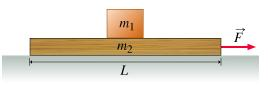# Problem: A small box of mass m1 is sitting on a board of mass m2 and length L. The board rests on a frictionless horizontal surface. The coefficient of static friction between the board and the box is μs. The coefficient of kinetic friction between the board and the box is, as usual, less than μs.Throughout the problem, use g for the magnitude of the acceleration due to gravity. In the hints, use Ff for the magnitude of the friction force between the board and the box.Find Fmin, the constant force with the least magnitude that must be applied to the board in order to pull the board out from under the the box (which will then fall off of the opposite end of the board).Express your answer in terms of some or all of the variables mus, m1, m2, g, and L. Do not include Ff in your answer.

###### FREE Expert Solution

In this problem, the surface is frictionless.

The frictional force, Fr is acting between the board and the box.

We also need to note that the system is being accelerated from the applied force.

The box will experience a force that acts against friction, given by:

$\overline{){{\mathbf{F}}}_{{\mathbf{r}}}{\mathbf{=}}{{\mathbf{m}}}_{{\mathbf{1}}}{\mathbf{a}}}$

80% (253 ratings)###### Problem Details

A small box of mass m1 is sitting on a board of mass m2 and length L. The board rests on a frictionless horizontal surface. The coefficient of static friction between the board and the box is μs. The coefficient of kinetic friction between the board and the box is, as usual, less than μs.

Throughout the problem, use g for the magnitude of the acceleration due to gravity. In the hints, use Ff for the magnitude of the friction force between the board and the box.Find Fmin, the constant force with the least magnitude that must be applied to the board in order to pull the board out from under the the box (which will then fall off of the opposite end of the board).

Express your answer in terms of some or all of the variables mus, m1, m2, g, and L. Do not include Ff in your answer.

Frequently Asked Questions

What scientific concept do you need to know in order to solve this problem?

Our tutors have indicated that to solve this problem you will need to apply the Systems of Objects with Friction concept. You can view video lessons to learn Systems of Objects with Friction. Or if you need more Systems of Objects with Friction practice, you can also practice Systems of Objects with Friction practice problems.

What professor is this problem relevant for?

Based on our data, we think this problem is relevant for Professor Zakhidov's class at UTD.